# Preface

This section gives soem examples of plotting cycloids because they appear as solutions of some differential equations.

# Cycloids

A cycloid is the curve traced by a point on the rim of a circular wheele, of radius 𝑎 rolling along a straight line. It was studied and named by Galileo in 1599. However, mathematical historian Paul Tannery cited the Syrian philosopher Iamblichus as evidence that the curve was likely known in antiquity. The history of cycloid was prepared by Tom Roidt. Its curve can be generalized by choosing a point not on the rim, but at any distance b from the center on a fixed radius. Alternatively, if we assume that the circle is turning at a constant rate, the parameter t could also be regarded as measuring the elapsed time since the circle began rolling. We will call the radius of our circle "𝑎." A graph of the cycloid curve and its generating circle, is presented beow. If b=𝑎, we get a usual cycloid.

cycloid[a_, t_] := {a*t - a*Sin[t], a - a*Cos[t]}
axes[x_, y_, f_, a_] :=
Arrow[{{0, 0}, #}] & /@ {{x, 0}, {0, y}}, {Text[
Style["x", FontSize -> Scaled[f]], {0.9*x, .15*y}],
Text[Style["y", FontSize -> Scaled[f]], {0.07 x, 1*y}]}]]
data1 = Table[{t, cycloid[1, t][], cycloid[1, t][]}, {t, 0, 2 $Pi], 0.01}]; Show[ListLinePlot[{#2, #3} & @@@ data1, PlotRange -> {{-\[Pi]/4, 3 \[Pi]}, {0, 3}}, AspectRatio -> Automatic, Ticks -> {None, None}, Frame -> False, PlotRange -> All, PlotRangeClipping -> False, ImagePadding -> {{20, 20}, {20, 20}}], axes[9.7, 3.2, .07, .07], Graphics[{{Dashed, Circle[{0, 1}, 1]}, Point[{\[Pi] + .5, 1}], Point[{4.123, 1.9}], Circle[{\[Pi] + .5, 1}, 1], Line[{{0, 0}, {0, 1}}], Point[{0, 0}], Point[{\[Pi]*2, 1}], Point[{\[Pi]*2, 0}], Point[{0, 1}], {Dashed, Circle[{\[Pi]*2, 1}, 1]}, Line[{{\[Pi]*2, 0}, {\[Pi]*2, 1}}], Line[{{\[Pi] + .5, 1}, {4.123, 1.9}}]}]]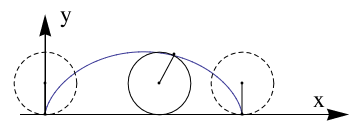Another simple plot: ParametricPlot[{t - 2*Sin[t], 1 - 2*Cos[t]}, {t, 0, 3 Pi}, AxesLabel -> {"x", "y"}, PlotLabel -> "Cycloid", AspectRatio -> Automatic]Another version: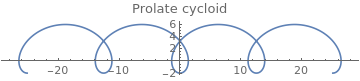Clear[f, g, theta]; f[theta_] = 2 theta - 4 Sin[theta]; g[theta_] = 2 - 4 Cos[theta]; ParametricPlot[{f[theta], g[theta]}, {theta, -4*Pi, 4*Pi}, PlotLabel ->"Prolate cycloid"] Now we plot cycloid downward: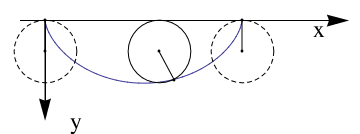Graphics[Join[{Arrowheads[a]}, Arrow[{{0, 0}, #}] & /@ {{x, 0}, {0, y}}, {Text[ Style["x", FontSize -> Scaled[f]], {0.9*x, .08*y}], Text[Style["y", FontSize -> Scaled[f]], {0.1 x, 1*y}]}]] data1 = Table[{t, cycloid[1, 1][t][], cycloid[1, 1][t][]}, {t, 0, 2 \[Pi], 0.01}]; Show[ListLinePlot[{#2, -#3} & @@@ data1, PlotRange -> {{-\[Pi]/4, 3 \[Pi]}, {-3, 0}}, AspectRatio -> Automatic, Ticks -> {None, None}, Frame -> False, PlotRange -> All, PlotRangeClipping -> False, ImagePadding -> {{20, 20}, {20, 20}}], axes[9.7, -3.2, .07, .07], Graphics[{{Dashed, Circle[{0, -1}, 1]}, Point[{\[Pi] + .5, -1}], Point[{4.123, -1.9}], Circle[{\[Pi] + .5, -1}, 1], Line[{{0, 0}, {0, -1}}], Point[{0, 0}], Point[{\[Pi]*2, -1}], Point[{\[Pi]*2, 0}], Point[{0, -1}], {Dashed, Circle[{\[Pi]*2, -1}, 1]}, Line[{{\[Pi]*2, 0}, {\[Pi]*2, -1}}], Line[{{\[Pi] + .5, -1}, {4.123, -1.9}}]}] Now we plot orthogonal curves to cycloid: axes[x_, y_, f_, a_] := Graphics[Join[{Arrowheads[a]}, Arrow[{{0, 0}, #}] & /@ {{x, 0}, {0, y}}, {Text[ Style["x", FontSize -> Scaled[f]], {0.9*x, .08*y}], Text[Style["y", FontSize -> Scaled[f]], {0.1 x, 1*y}]}]] data1 = Table[{\[Tau], Cycloid[1, \[Tau]][], Cycloid[1, \[Tau]][]}, {\[Tau], 0, 2 \[Pi], 0.01}]; data2 = Table[{\[Tau], Cycloid[1.5, \[Tau]][], Cycloid[1.5, \[Tau]][]}, {\[Tau], 0, 3.14^2 - 0.6, 0.01}]; data1a = Table[{\[Rho], Cycloid[\[Rho], 2][], Cycloid[\[Rho], 2][]}, {\[Rho], .3, 10, .01}]; data2a = Table[{\[Rho], Cycloid[\[Rho], 3][], Cycloid[\[Rho], 3][]}, {\[Rho], .5, 10, .01}]; data3a = Table[{\[Rho], Cycloid[\[Rho], 4][], Cycloid[\[Rho], 4][]}, {\[Rho], .65, 10, .01}]; Show[ListLinePlot[{#2, -#3} & @@@ data1, AspectRatio -> Automatic, PlotRange -> {{-\[Pi]/4, 5*\[Pi]}, {-5, 0}}, PlotStyle -> {Black, Thick}, Ticks -> {None, None}, Frame -> False, PlotRange -> All, PlotRangeClipping -> False, ImagePadding -> {{20, 20}, {20, 20}}], ListLinePlot[{#2, -#3} & @@@ data2, PlotStyle -> {Black, Thick}, PlotRange -> {{-\[Pi]/4, 2*\[Pi] + \[Pi]/4}, {-5, 0}}, Ticks -> {None, None}, Frame -> False, PlotRange -> All, PlotRangeClipping -> False, ImagePadding -> {{20, 20}, {20, 20}}], ListLinePlot[{#2, -#3} & @@@ data1a, PlotRange -> {{-\[Pi]/4, 2*\[Pi] + \[Pi]/4}, {-5, 0}}, Ticks -> {None, None}, Frame -> False, PlotRange -> All, PlotStyle -> {Blue}, PlotRangeClipping -> False, ImagePadding -> {{20, 20}, {20, 20}}], ListLinePlot[{#2, -#3} & @@@ data2a, PlotRange -> {{-\[Pi]/4, 2*\[Pi] + \[Pi]/4}, {-5, 0}}, Ticks -> {None, None}, Frame -> False, PlotRange -> All, PlotStyle -> {Blue}, PlotRangeClipping -> False, ImagePadding -> {{20, 20}, {20, 20}}], ListLinePlot[{#2, -#3} & @@@ data3a, PlotRange -> {{-\[Pi]/4, 2*\[Pi] + \[Pi]/4}, {-5, 0}}, Ticks -> {None, None}, Frame -> False, PlotStyle -> {Blue}, PlotRange -> All, PlotRangeClipping -> False, ImagePadding -> {{20, 20}, {20, 20}}], axes[16.5, -5.2, .07, .07]]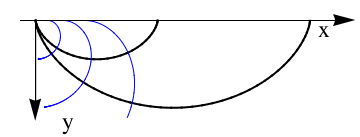Trochoid A trochoid (from the Greek word for wheel, "trochos") is a roulette formed by a circle rolling along a line. In other words, it is the curve traced out by a point fixed to a circle (where the point may be on, inside, or outside the circle) as it rolls along a straight line. The Parametrization of the trochoid can be made through the following equations: \[ x = at-b\,\sin t , \qquad y=a-b\,\cos t$trochoid[a_, b_][t_] := {a*t - b*Sin[t], a - b*Cos[t]}
Manipulate[
ParametricPlot[
trochoid[a, b][t] // Evaluate, {t, -$Pi]/2, 5*\[Pi]/2}], {a, 1, 5}, {b, 1, 5}]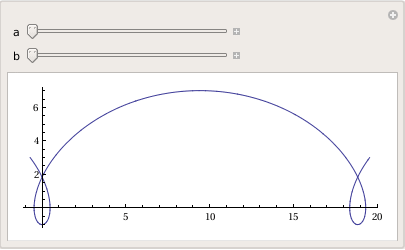Cycloid[\[Rho]_, \[Tau]_] := {\[Rho]*\[Tau] - \[Rho]^2* Sin[\[Tau]/\[Rho]], \[Rho]^2*(1 - Cos[\[Tau]/\[Rho]])}; PolarPlot[Cycloid[1.5,theta],{theta, 0, 4*Pi}]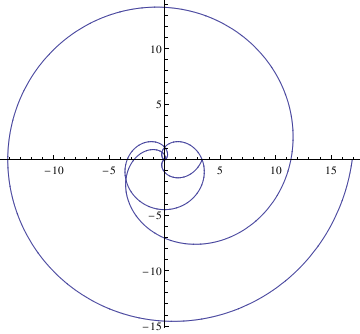Hypocycloid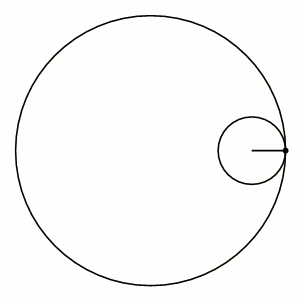Hypocycloid If the smaller circle has radius r, and the larger circle has radius R = kr, then the parametric equations for the curve can be given by either: \[ \begin{split} x(\theta ) &= \left( R-r \right) \cos \theta + r\,\cos \left( \frac{R-r}{r}\,\theta \right) , \\ y(\theta ) &= \left( R-r \right) \sin \theta - r\,\sin \left( \frac{R-r}{r}\,\theta \right) , \end{split}$
or
$\begin{split} x(\theta ) &= r \left( k-1 \right) \cos\theta + r\,\cos \left[ \left( k-1 \right) \theta \right] , \\ y(\theta ) &= r \left( k-1 \right) \sin\theta - r\,\sin \left[ \left( k-1 \right) \theta \right] . \end{split}$
If k is an integer, then the curve is closed, and has k cusps (i.e., sharp corners, where the curve is not differentiable). Specially for k=2 the curve is a straight line and the circles are called Cardano circles. Girolamo Cardano (1501--1576) was the first to describe these hypocycloids and their applications to high-speed printing.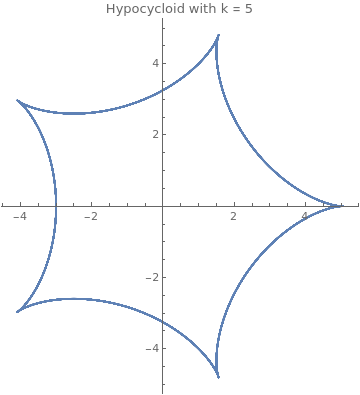Astroid k = 5 ParametricPlot[{4*Cos[theta] + Cos[4*theta], 4*Sin[theta] - Sin[4*theta]}, {theta, -4*Pi, 8*Pi}, PlotLabel -> "Hypocycloid"]

If k is a rational number, say k = p/q expressed in simplest terms, then the curve has p cusps.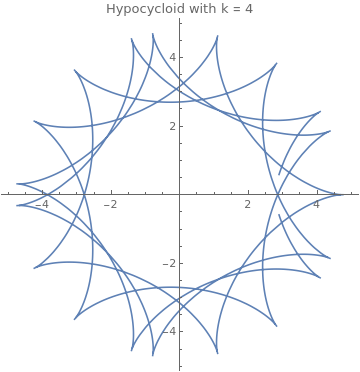Astroid k = 4.7 ParametricPlot[{3.7*Cos[theta] + Cos[3.7*theta], 3.7*Sin[theta] - Sin[3.7*theta]}, {theta, -4*Pi, 4*Pi}, PlotLabel -> "Hypocycloid with k = 4.7"]

If k is an irrational number, then the curve never closes, and fills the space between the larger circle and a circle of radius R − 2r.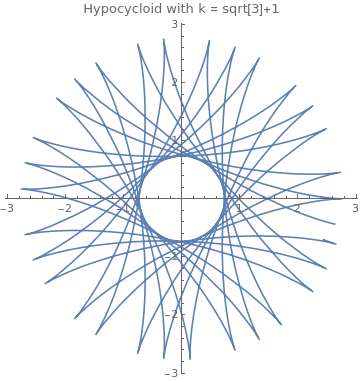Astroid k = Sqrt+1 ParametricPlot[{Sqrt*Cos[theta] + Cos[Sqrt*theta], Sqrt*Sin[theta] - Sin[Sqrt*theta]}, {theta, -8*Pi, 14*Pi}, PlotLabel -> "Hypocycloid with k = 4.7"]

Epitrochoid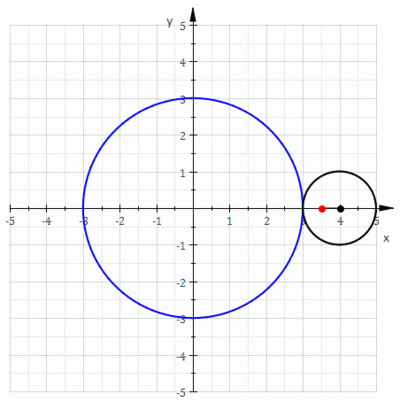Epitrochoid An epitrochoid is a roulette traced by a point attached to a circle of radius r rolling around the outside of a fixed circle of radius R, where the point is at a distance d from the center of the exterior circle. The parametric equations for an epitrochoid are
$\begin{split} x(\theta ) &= \left( R+r \right) \cos \theta - d\,\cos \left( \frac{R+r}{r}\,\theta \right) , \\ y(\theta ) &= \left( R+r \right) \sin \theta - d\,\sin \left( \frac{R+r}{r}\,\theta \right) , \end{split}$
where θ is a parameter (not the polar angle). Special cases include the limaçon with R = r and the epicycloid with d = r.